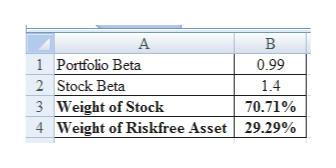# If a portfolio of the two assets has a beta of .99, what are the portfolio weights? (Do not round intermediate calculations and round your answers to 2 decimal places, e.g., 32.16.)   Weight of stock Risk-free weight

Question
1 views

If a portfolio of the two assets has a beta of .99, what are the portfolio weights? (Do not round intermediate calculations and round your answers to 2 decimal places, e.g., 32.16.)

 Weight of stock Risk-free weight
check_circle

Step 1

Hi. Since there is no information given regarding the beta of stock, we are randomly assuming the beta as 1.40. Kindly replace with the original beta values given.

Step 2

Calculation of Portfolio Weights:

The weight of stock is 70.71% and the weight of risk-free asset is 29.29%.

...help_outlineImage TranscriptioncloseA В 1 Portfolio Beta 0.99 2 Stock Beta 1.4 Weight of Stock Weight of Riskfree Asset 3 70.71% 4 29.29% fullscreen

### Want to see the full answer?

See Solution

#### Want to see this answer and more?

Solutions are written by subject experts who are available 24/7. Questions are typically answered within 1 hour.*

See Solution
*Response times may vary by subject and question.
Tagged in